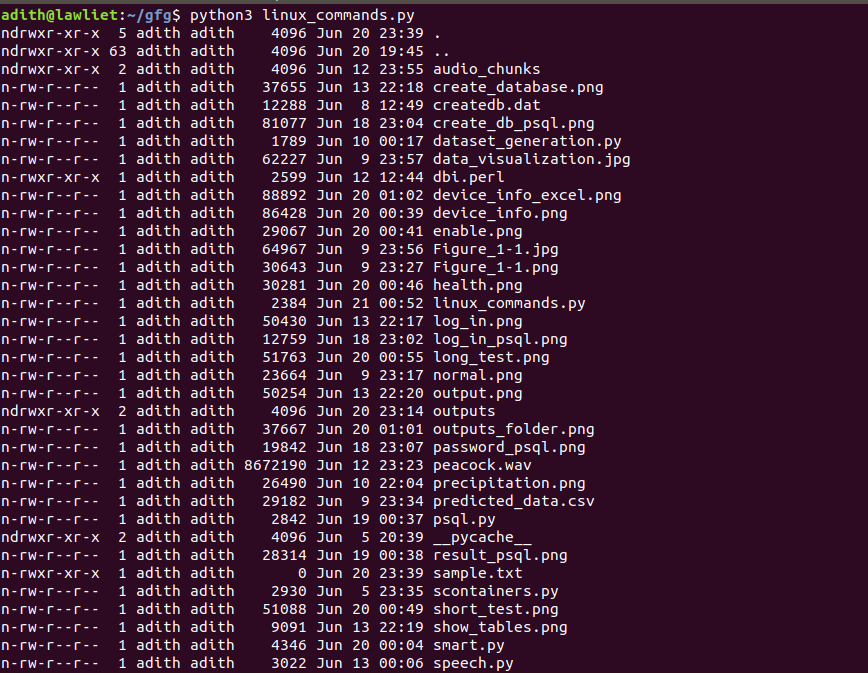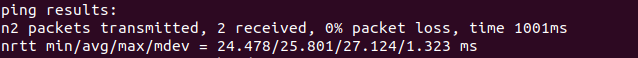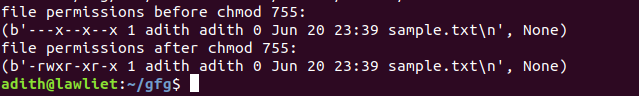# Python | Execute and parse Linux commands

• Difficulty Level : Medium
• Last Updated : 25 Jun, 2019

Prerequisite: Introduction to Linux Shell and Shell Scripting

Linux is one of the most popular operating systems and is a common choice for developers. It is popular because it is open source, it’s free and customizable, it is very robust and adaptable.

An operating system mainly consists of two parts: The kernel and the Shell. The kernel basically handles communication between the software and the hardware. The shell takes inputs or commands from the user and produces an output. Most Linux distributions nowadays use the BASH shell (Bourne again shell). Shell commands and scripts are very powerful and are used commonly by developers.

In this article, we shall look at executing and parsing Linux commands using python.

### Subprocess –

Subprocess is a module in Python that allows us to start new applications or processes in Python. This module intends to replace several older modules in python. We can use this module to run other programs or execute Linux commands.

### Starting a process –

A new process can be spawned by using the Popen function defined in the subprocess module. It is a constructor for the Popen class that takes arguments to set up the new process. The underlying process creation and management in this module is handled by the Popen class.

Arguments:

1. The first parameter is a list that contains the commands and their options if any.
ex: `['ls', '-l']`
the above example is equivalent to typing ‘ls -l’ in the terminal

2. The second parameter is the stdout value. it specifies the standard output.
ex: `stdout = subprocess.PIPE`
This indicates that a new pipe or redirection should be created. The default value is
“None”, which means that no redirection will occur.

We can retrieve the output of a command by using the communicate function. It reads data from stdout and stderr until it reaches the end-of-file and waits for the process to terminate. It returns a tuple that contains the output data and the error if any.

Syntax:

````data = subprocess.Popen(['ls', '-l', filename], stdout = subprocess.PIPE)`
`output = data.communicate()````

The output of the executed command is stored in data. Using these functions, we can execute Linux commands and fetch their output.

## Listing the directories –

We can use the ‘ls’ command with options such as ‘-l’, ‘-al’, etc to list all the files in the current directory. We can then parse this output and print it in a presentable format. The `get_permissions()` function parses the output of the list command and retrieves only the names of the files and their corresponding permissions.

 `# importing libraries``import` `subprocess``import` `os`` ` `# a function to list the files in``# the current directory and ``# parse the output.``def` `list_command(args ``=` `'-l'``):`` ` `    ``# the ls command``    ``cmd ``=` `'ls'`` ` `    ``# using the Popen function to execute the``    ``# command and store the result in temp.``    ``# it returns a tuple that contains the ``    ``# data and the error if any.``    ``temp ``=` `subprocess.Popen([cmd, args], stdout ``=` `subprocess.PIPE)``     ` `    ``# we use the communicate function``    ``# to fetch the output``    ``output ``=` `str``(temp.communicate())``     ` `    ``# splitting the output so that``    ``# we can parse them line by line``    ``output ``=` `output.split(``"\n"``)``     ` `    ``output ``=` `output[``0``].split(``'\\'``)`` ` `    ``# a variable to store the output``    ``res ``=` `[]`` ` `    ``# iterate through the output``    ``# line by line``    ``for` `line ``in` `output:``        ``res.append(line)`` ` `    ``# print the output``    ``for` `i ``in` `range``(``1``, ``len``(res) ``-` `1``):``        ``print``(res[i])`` ` `    ``return` `res`` ` `# parse the output of the ls ``# command and fetch the permissions``# of the files and store them in ``# a text file .``def` `get_permissions():`` ` `    ``# get the output of the ``    ``# list command``    ``res ``=` `list_command(``'-l'``)`` ` `    ``permissions ``=` `{}``     ` `    ``# iterate through all the rows``    ``# and retrieve the name of the file``    ``# and its permission.``    ``for` `i ``in` `range``(``1``, ``len``(res) ``-` `1``):``        ``line ``=` `res[i]`` ` `        ``line ``=` `line.split(``' '``)`` ` `        ``folder_name ``=` `line[``len``(line) ``-` `1``]``        ``permission_value ``=` `line[``0``]`` ` `        ``permissions[folder_name] ``=` `permission_value`` ` `    ``# create a directory called``    ``# outputs to store the output files``    ``try``:``        ``os.mkdir(``'outputs'``)`` ` `    ``except``:`` ` `        ``pass`` ` `    ``os.chdir(``'outputs'``)`` ` `    ``# open the output file``    ``out ``=` `open``(``'permissions.txt'``, ``'w'``)`` ` `    ``out.write(``'Folder Name   Permissions\n\n'``)`` ` `    ``# write to the output file``    ``for` `folder ``in` `permissions:`` ` `        ``out.write(folder ``+` `' : '` `+` `permissions[folder] ``+` `'\n'``)`` ` `    ``os.chdir(``'..'``)``    ``return` `permissions`` ` `if` `__name__ ``=``=` `'__main__'``:``    ``list_command(``'-al'``)`

Output :## Ping command –

The ping command stands for Packet Internet Groper. It is most commonly used to check the connectivity between two systems or nodes. Using the ping command, we can check whether the connection between one node and another is healthy or not. It exchanges packets of data between two nodes and also calculates the round trip time.

 `# importing libraries``import` `subprocess``import` `os`` ` `# a function to ping given host``def` `ping(host):`` ` `    ``# command is pong``    ``cmd ``=` `'ping'`` ` `    ``# send two packets of data to the host``    ``# this is specified by '-c 2' in the ``    ``# args list``    ``temp ``=` `subprocess.Popen([cmd, ``'-c 2'``, host], stdout ``=` `subprocess.PIPE)``     ` `    ``# get the output of ping``    ``output ``=` `str``(temp.communicate())``         ` `    ``output ``=` `output.split(``"\n"``)``     ` `    ``output ``=` `output[``0``].split(``'\\'``)`` ` `    ``# variable to store the result``    ``res ``=` `[]`` ` `    ``for` `line ``in` `output:``        ``res.append(line)`` ` `    ``# print the results``    ``print``(``'ping results: '``)``    ``print``(``'\n'``.join(res[``len``(res) ``-` `3``:``len``(res) ``-` `1``]))`` ` `    ``return` `res`` ` ` ` `if` `__name__ ``=``=` `'__main__'``:``         ` `    ``# ping google``    ``ping(``'www.google.com'``)`

Output :## Changing permissions –

The `chmod `command can be used to change file permissions. It is an abbreviation of change mode. More info can be found here

 `# importing libraries``import` `subprocess``import` `os`` ` ` ` `# functio to change the permissions``# of a given file``def` `change_permissions(args, filename):`` ` `    ``# command name``    ``cmd ``=` `'chmod'`` ` `    ``# getting the permissions of``    ``# the file before chmod``    ``ls ``=` `'ls'`` ` `    ``data ``=` `subprocess.Popen([ls, ``'-l'``, filename], stdout ``=` `subprocess.PIPE)`` ` `    ``output ``=` `str``(data.communicate())`` ` `    ``print``(``'file permissions before chmod % s: '` `%``(args))``    ``print``(output)`` ` `    ``# executing chmod on the specified file``    ``temp ``=` `subprocess.Popen([cmd, args, filename], stdout ``=` `subprocess.PIPE)`` ` `    ``# getting the permissions of the ``    ``# file after chmod``    ``data ``=` `subprocess.Popen([ls, ``'-l'``, filename], stdout ``=` `subprocess.PIPE)`` ` `    ``output ``=` `str``(data.communicate())`` ` `    ``# printing the permissions after chmod``    ``print``(``'file permissions after chmod % s: '` `%``(args))``    ``print``(output)`` ` `if` `__name__ ``=``=` `'__main__'``:``         ` `    ``# changing the permissions of 'sample.txt' ``    ``change_permissions(``'755'``, ``'sample.txt'``)`

Output :My Personal Notes arrow_drop_up